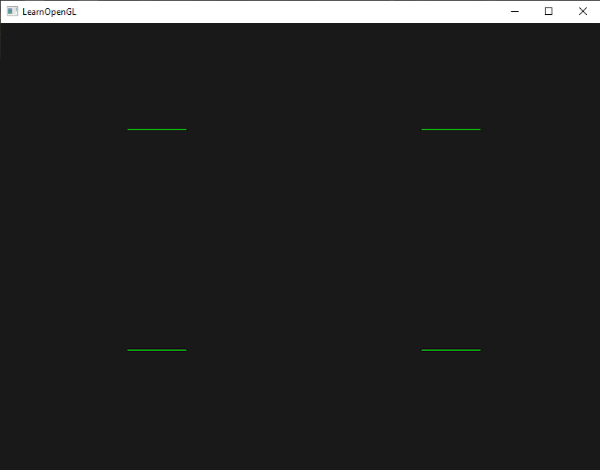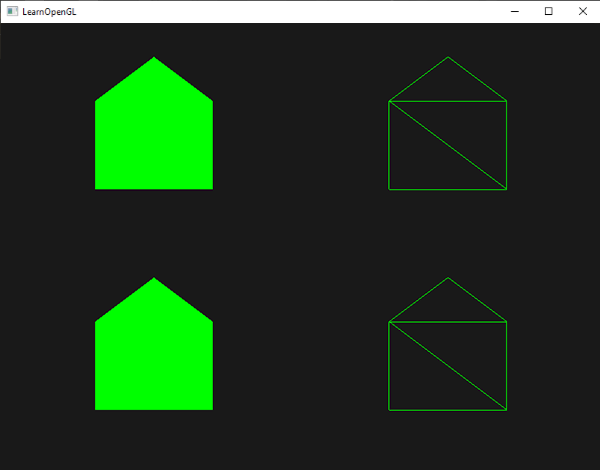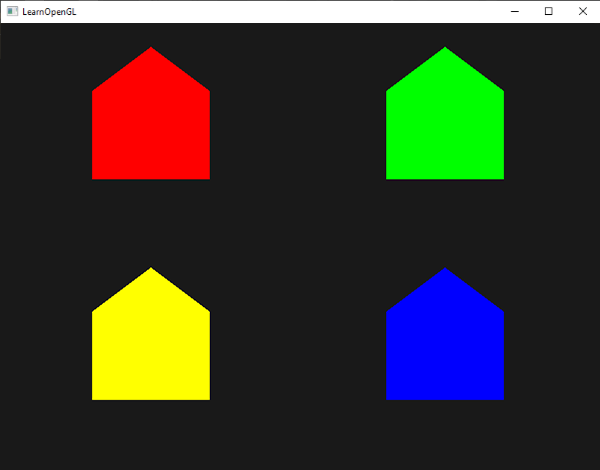# 几何着色器

#version 330 core
layout (points) in;
layout (line_strip, max_vertices = 2) out;

void main() {
gl_Position = gl_in.gl_Position + vec4(-0.1, 0.0, 0.0, 0.0);
EmitVertex();

gl_Position = gl_in.gl_Position + vec4(0.1, 0.0, 0.0, 0.0);
EmitVertex();

EndPrimitive();
}


points 绘制GL_POINTS基本图形的时候（1）
lines 当绘制GL_LINES或GL_LINE_STRIP（2）时
triangles GL_TRIANGLES, GL_TRIANGLE_STRIP或GL_TRIANGLE_FAN（3）

• points
• line_strip
• triangle_stripin gl_Vertex
{
vec4 gl_Position;
float gl_PointSize;
float gl_ClipDistance[];
} gl_in[];



void main() {
gl_Position = gl_in.gl_Position + vec4(-0.1, 0.0, 0.0, 0.0);
EmitVertex();

gl_Position = gl_in.gl_Position + vec4(0.1, 0.0, 0.0, 0.0);
EmitVertex();

EndPrimitive();
}glDrawArrays(GL_POINTS, 0, 4);


## 使用几何着色器

GLfloat points[] = {
-0.5f,  0.5f, // 左上方
0.5f,  0.5f,  // 右上方
0.5f, -0.5f,  // 右下方
-0.5f, -0.5f  // 左下方
};


#version 330 core
layout (location = 0) in vec2 position;

void main()
{
gl_Position = vec4(position.x, position.y, 0.0f, 1.0f);
}


#version 330 core
out vec4 color;

void main()
{
color = vec4(0.0f, 1.0f, 0.0f, 1.0f);
}


shader.Use();
glBindVertexArray(VAO);
glDrawArrays(GL_POINTS, 0, 4);
glBindVertexArray(0);#version 330 core
layout (points) in;
layout (points, max_vertices = 1) out;

void main() {
gl_Position = gl_in.gl_Position;
EmitVertex();
EndPrimitive();
}


geometryShader = glCreateShader(GL_GEOMETRY_SHADER);
...## 造几个房子#version 330 core
layout (points) in;
layout (triangle_strip, max_vertices = 5) out;

void build_house(vec4 position)
{
gl_Position = position + vec4(-0.2f, -0.2f, 0.0f, 0.0f);// 1:左下角
EmitVertex();
gl_Position = position + vec4( 0.2f, -0.2f, 0.0f, 0.0f);// 2:右下角
EmitVertex();
gl_Position = position + vec4(-0.2f,  0.2f, 0.0f, 0.0f);// 3:左上
EmitVertex();
gl_Position = position + vec4( 0.2f,  0.2f, 0.0f, 0.0f);// 4:右上
EmitVertex();
gl_Position = position + vec4( 0.0f,  0.4f, 0.0f, 0.0f);// 5:屋顶
EmitVertex();
EndPrimitive();
}

void main()
{
build_house(gl_in.gl_Position);
}GLfloat points[] = {
-0.5f,  0.5f, 1.0f, 0.0f, 0.0f, // 左上
0.5f,  0.5f, 0.0f, 1.0f, 0.0f, // 右上
0.5f, -0.5f, 0.0f, 0.0f, 1.0f, // 右下
-0.5f, -0.5f, 1.0f, 1.0f, 0.0f  // 左下
};


#version 330 core
layout (location = 0) in vec2 position;
layout (location = 1) in vec3 color;

out VS_OUT {
vec3 color;
} vs_out;

void main()
{
gl_Position = vec4(position.x, position.y, 0.0f, 1.0f);
vs_out.color = color;
}


in VS_OUT {
vec3 color;
} gs_in[];


Important

in vec3 vColor[];

out vec3 fColor;


fColor = gs_in.color; //只有一个输出颜色，所以直接设置为gs_in
gl_Position = position + vec4(-0.2f, -0.2f, 0.0f, 0.0f);    // 1:左下
EmitVertex();
gl_Position = position + vec4( 0.2f, -0.2f, 0.0f, 0.0f);    // 2:右下
EmitVertex();
gl_Position = position + vec4(-0.2f,  0.2f, 0.0f, 0.0f);    // 3:左上
EmitVertex();
gl_Position = position + vec4( 0.2f,  0.2f, 0.0f, 0.0f);    // 4:右上
EmitVertex();
gl_Position = position + vec4( 0.0f,  0.4f, 0.0f, 0.0f);    // 5:屋顶
EmitVertex();
EndPrimitive();fColor = gs_in.color;
gl_Position = position + vec4(-0.2f, -0.2f, 0.0f, 0.0f);
EmitVertex();
gl_Position = position + vec4( 0.2f, -0.2f, 0.0f, 0.0f);
EmitVertex();
gl_Position = position + vec4(-0.2f,  0.2f, 0.0f, 0.0f);
EmitVertex();
gl_Position = position + vec4( 0.2f,  0.2f, 0.0f, 0.0f);
EmitVertex();
gl_Position = position + vec4( 0.0f,  0.4f, 0.0f, 0.0f);
fColor = vec3(1.0f, 1.0f, 1.0f);
EmitVertex();
EndPrimitive();# 爆破物体vec3 GetNormal()
{
vec3 a = vec3(gl_in.gl_Position) - vec3(gl_in.gl_Position);
vec3 b = vec3(gl_in.gl_Position) - vec3(gl_in.gl_Position);
return normalize(cross(a, b));
}


vec4 explode(vec4 position, vec3 normal)
{
float magnitude = 2.0f;
vec3 direction = normal * ((sin(time) + 1.0f) / 2.0f) * magnitude;
return position + vec4(direction, 0.0f);
}


#version 330 core
layout (triangles) in;
layout (triangle_strip, max_vertices = 3) out;

in VS_OUT {
vec2 texCoords;
} gs_in[];

out vec2 TexCoords;

uniform float time;

vec4 explode(vec4 position, vec3 normal) { ... }

vec3 GetNormal() { ... }

void main() {
vec3 normal = GetNormal();

gl_Position = explode(gl_in.gl_Position, normal);
TexCoords = gs_in.texCoords;
EmitVertex();
gl_Position = explode(gl_in.gl_Position, normal);
TexCoords = gs_in.texCoords;
EmitVertex();
gl_Position = explode(gl_in.gl_Position, normal);
TexCoords = gs_in.texCoords;
EmitVertex();
EndPrimitive();
}


glUniform1f(glGetUniformLocation(shader.Program, "time"), glfwGetTime());


# 显示法向量

c++
DrawScene();
DrawScene();


#version 330 core
layout (location = 0) in vec3 position;
layout (location = 1) in vec3 normal;

out VS_OUT {
vec3 normal;
} vs_out;

uniform mat4 projection;
uniform mat4 view;
uniform mat4 model;

void main()
{
gl_Position = projection * view * model * vec4(position, 1.0f);
mat3 normalMatrix = mat3(transpose(inverse(view * model)));
vs_out.normal = normalize(vec3(projection * vec4(normalMatrix * normal, 1.0)));
}


#version 330 core
layout (triangles) in;
layout (line_strip, max_vertices = 6) out;

in VS_OUT {
vec3 normal;
} gs_in[];

const float MAGNITUDE = 0.4f;

void GenerateLine(int index)
{
gl_Position = gl_in[index].gl_Position;
EmitVertex();
gl_Position = gl_in[index].gl_Position + vec4(gs_in[index].normal, 0.0f) * MAGNITUDE;
EmitVertex();
EndPrimitive();
}

void main()
{
GenerateLine(0); // First vertex normal
GenerateLine(1); // Second vertex normal
GenerateLine(2); // Third vertex normal
}


#version 330 core
out vec4 color;

void main()
{
color = vec4(1.0f, 1.0f, 0.0f, 1.0f);
}